### Home > CCG > Chapter 3 > Lesson 3.2.4 > Problem3-93

3-93.

Examine the diagrams below. For each one, write and solve an equation to find $x$. Show all work.

1.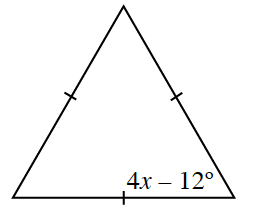Equilateral triangles have three congruent angles.

1.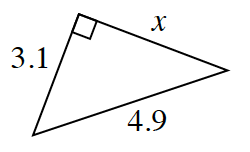Use the Pythagorean Theorem to solve for $x$.

1.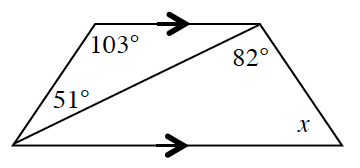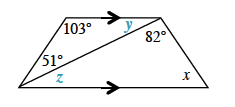$\begin{array}{l} ∠y=180º-103º-51º\\ ∠y=26° \end{array}$

Because $∠y$ and $∠z$ are alternate interior angles, they are congruent. Therefore, $x=180º-82º-26º$.

$x=72º$

1.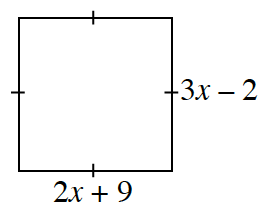$3x-2=2x+9$